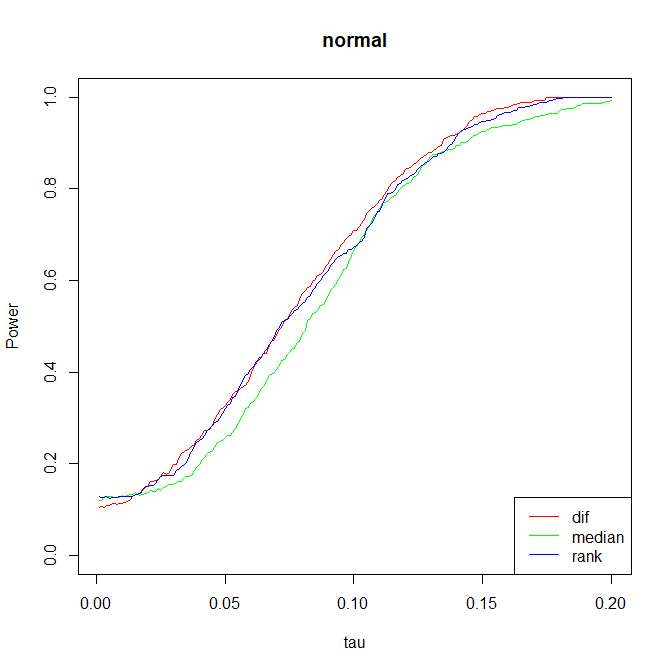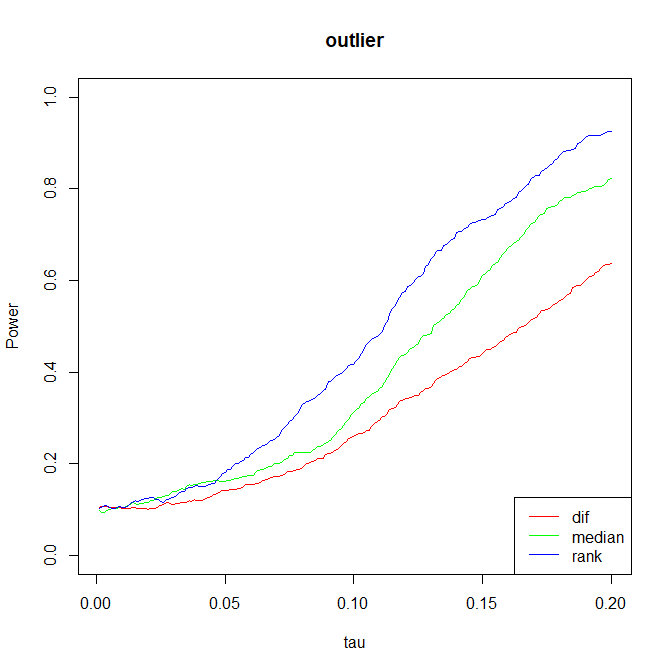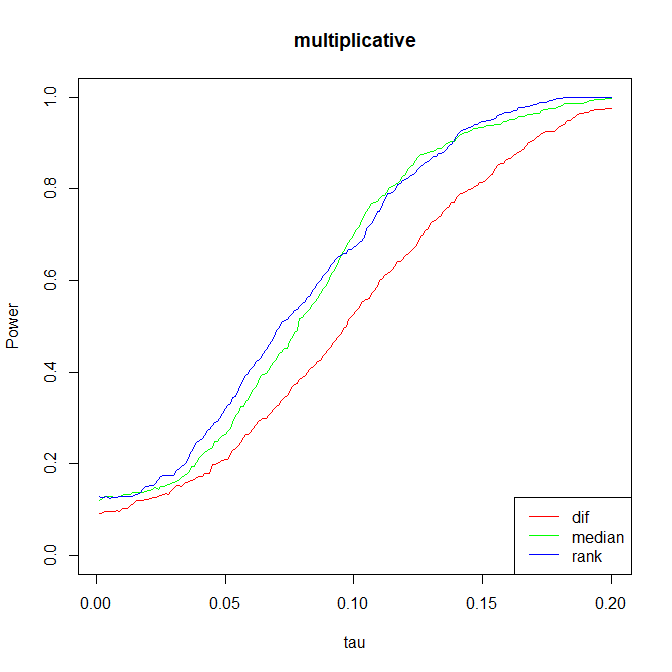## 使用的数据

### 试验后结果的分层累计分布

0 0.14 0.14 0.16 0.17
1 0.19 0.40 0.22 0.46
2 0.32 0.63 0.35 0.54
3 0.73 0.83 0.59 0.77
4 0.89 0.91 0.86 0.91
5 0.92 0.97 0.95 0.94
6 1.00 1.00 1.00 1.00

1 ? 3 1 4 3
2 ? 5 1 6 5
3 ? 0 1 4 0
4 4 ? 0 4 4
5 0 ? 0 1 0
6 1 ? 0 5 1

## 检验步骤

### 确定零假设(Fisher Sharp Null Hypothesis)

Fisher 当时提出的原假设为 $H_0: Y_i(0)=Y_i(1)$，但很自然的我们可以将它扩展，只要最后能确定所有的潜在结果即可，比如

• $H_0: Y_i(1)=Y_i(0)+C$
• $H_0: Y_i(1)=Y_i(0)*C$
• $H_0: Y_i(1)=Y_i(0)+C_i$

1 (3) 3 1 4 3 4
2 (5) 5 1 6 5 6
3 (0) 0 1 4 0 1.5
4 4 (4) 0 4 4 5
5 0 (0) 0 1 0 1.5
6 1 (1) 0 5 1 3

(注: rank 的计算方式即直接排序，然后分配123456。如果值相等则取平均数作为rank。如果将其都减去 $(N+1)/2$后，则其和为0。我们将平移后的结果记为 $R_i$)

### 选择统计量 $T$

$W_1$ $W_2$ $W_3$ $W_4$ $W_5$ $W_6$ $T_1$ $T_2$
0 0 0 1 1 1 -1.00 -0.67
0 0 1 0 1 1 -3.67 -3.00
0 0 1 1 0 1 -1.00 -0.67
0 0 1 1 1 0 -1.67 -1.67
0 1 0 0 1 1 -0.33 0.00
0 1 0 1 0 1 2.33 2.33
0 1 0 1 1 0 1.67 1.33
0 1 1 0 0 1 -0.33 0.00
0 1 1 0 1 0 -1.00 -1.00
0 1 1 1 0 0 1.67 1.33
1 0 0 0 1 1 -1.67 -1.33
1 0 0 1 0 1 1.00 1.00
1 0 0 1 1 0 0.33 0.00
1 0 1 0 0 1 -1.67 -1.33
1 0 1 0 1 0 -2.33 -2.33
1 1 1 1 0 0 0.33 0.00
1 1 0 0 0 1 1.67 1.67
1 1 0 0 1 0 1.00 0.67
1 1 0 1 0 0 3.67 3.00
1 1 1 0 0 0 1.00 0.67

• $T^\mathrm{dif}=\lvert \bar{Y}_t^\mathrm{obs} - \bar{Y}_c^\mathrm{obs}\rvert=\lvert\frac{\sum_{i:W_i=1}Y_i^\mathrm{obs}}{N_t}-\frac{\sum_{i:W_i=0}Y_i^\mathrm{obs}}{N_c}\rvert$
• $T^\mathrm{log}=\lvert\frac{\sum_{i:W_i=1}\ln(Y_i^\mathrm{obs})}{N_t}-\frac{\sum_{i:W_i=0}\ln(Y_i^\mathrm{obs})}{N_c}\rvert$
• $T^\mathrm{median}=\lvert \mathrm{med}_t(Y_i^{\mathrm{obs}})-\mathrm{med}_c(Y_i^{\mathrm{obs}})\rvert$
• $T^\mathrm{t-stat}=\lvert \frac{\bar{Y}_t^\mathrm{obs} - \bar{Y}_c^\mathrm{obs}}{\sqrt{s_c^2/N_c+s_t^2/N_t}}\rvert,\,s_t^2=\sum_{i:W_i=1}(Y_i^\mathrm{obs}-\bar{Y}_t^\mathrm{obs})^2/(N_t-1)$$s_c^2$ 类似
• $T^\mathrm{quant}=\lvert q_{\delta,t}(Y_i^{\mathrm{obs}})-q_{\delta,t}(Y_i^{\mathrm{obs}})\rvert$, $\delta_{q,c}$ 是经验累计分布 (e.c.d.f) 的 $\delta$ 分位数
• $T^\mathrm{rank}=\lvert \bar{R}_t - \bar{R}_c\rvert=\lvert\frac{\sum_{i:W_i=1}\bar{R}_i}{N_t}-\frac{\sum_{i:W_i=0}\bar{R}_i}{N_c}\rvert\rvert$

• $Y_i(0)\sim\mathcal{N}(\mu_c,\sigma_c^2),\,Y_i(1)\sim\mathcal{N}(\mu_t,\sigma_t^2)$

$T^{\mathrm{model}}=\lvert\widehat{\mu}_t-\widehat{\mu}_c\rvert=\lvert\bar{Y}_t^\mathrm{obs} - \bar{Y}_c^\mathrm{obs}\rvert=T^\mathrm{dif}$

• $\log Y_i(0)\sim\mathcal{N}(\mu_c,\sigma_c^2),\,\log Y_i(1)\sim\mathcal{N}(\mu_t,\sigma_t^2)$

$T^{\mathrm{model}}=\lvert\widehat{\mu}_{mle,t}-\widehat{\mu}_{mle,c}\rvert$

• 还可以用非参数的模型，如 Kolmogorov-Smirnov Statistic

$T^{\mathrm{ks}}=\sup\limits_y\lvert\widehat{F}_t(y)-\widehat{F}_c(y)\rvert=\max\limits_{i=1,\ldots,N}\lvert\widehat{F}_t(Y_i^\mathrm{obs})-\widehat{F}_c(Y_i^\mathrm{obs})\rvert$

其中 $\widehat{F}_c(y)=\frac{1}{N_c}\sum_{i:W_i=0}\boldsymbol{1}_{Y_i^\mathrm{obs}\leq y}$ ，即经验累计分布函数 (e.c.d.f)。$\widehat{F}_t(y)$ 类似

• 实验一：$Y_i(0)\sim\mathcal{N}(0,1),\,Y_i(1)=Y_i(0)+\tau$
• 实验二：在上一个实验的基础上，给 $20\%$ 的数据加上了 $5$ 的偏置使其变为离群值
• 实验三：在第一个实验的基础上，都取$\exp$，即 $Y_i(0)\sim\log-\mathcal{N}(0,1),\,Y_i(1)=Y_i(0)\cdot\exp{\tau}$### 带协变量的检验

$T^\mathrm{gain}=\frac{\sum_{i:W_i=1}(Y_i^\mathrm{obs}-X_i)}{N_t}-\frac{\sum_{i:W_i=0}(Y_i^\mathrm{obs}-X_i)}{N_c}=\bar{Y}_t^{\mathrm{obs}}-\bar{Y}_c^{\mathrm{obs}}-(\bar{X}_t-\bar{X}_c)$

$T^\mathrm{prop-change}=\frac{1}{N_t}\sum\limits_{i:W_i=1}\frac{Y_i^\mathrm{obs}-X_i}{X_i}-\frac{1}{N_c}\sum\limits_{i:W_i=0}\frac{Y_i^\mathrm{obs}-X_i}{X_i}$

$(\widehat{\beta}_0,\widehat{\beta}_X,\widehat{\beta}_W)=\arg\min\limits_{\widehat{\beta}_0,\widehat{\beta}_X,\widehat{\beta}_W}\sum\limits_{i=1}^{N}(Y_i^\mathrm{obs}-\widehat{\beta}_0-\widehat{\beta}_X\cdot X_i-\widehat{\beta}_W\cdot W_i)^2$

## 局限

Fisher Exact Test 是一个十分直观且简单的检验方法，但也带来了他的局限性，主要体现在两点

• Sharp null hypothesis 带来了太多的限制
• 拒绝了原假设后，对于组间效应不能给出一个预测量，连点估计都无法给出。